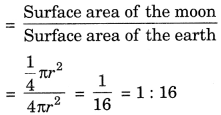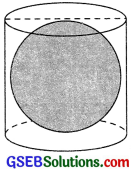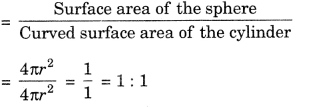# GSEB Solutions Class 9 Maths Chapter 13 Surface Areas and Volumes Ex 13.4

Gujarat Board GSEB Solutions Class 9 Maths Chapter 13 Surface Areas and Volumes Ex 13.4 Textbook Questions and Answers.

## Gujarat Board Textbook Solutions Class 9 Maths Chapter 13 Surface Areas and Volumes Ex 13.4

Question 1.
Find the surface area of a sphere of radius:
1. 10.5 cm
2. 5.6 cm
3. 14 cm
Solution:
1. r = 10.5 cm
∴ Surface area = 41πr2
= 4 x $$\frac {22}{7}$$ x (10.5)2
= 1386 cm2

2. r = 5.6 cm
∴ Surface area = 4m2
= 4x $$\frac {22}{7}$$ x (5.6)2
= 394.24 cm23. r = 14 cm
∴ Surface area = 4πr2
= 4 x $$\frac {22}{7}$$ x (14)2 = 2464 cm2

Question 2.
Find the surface area of a sphere of diameter:
1. 14 cm
2. 21 cm
3. 3.5 m
Solution:
1. Diameter = 14 cm
∴ Radius (r) = $$\frac {14}{2}$$ cm = 7 cm
∴ Surface area = 4πr2
= 4 x $$\frac {22}{7}$$ x (7)2 = 616 cm2

2. Diameter = 21 cm
∴ Radius (r) = $$\frac {21}{2}$$cm
∴ Surface area = 4πr2
= 4 x $$\frac {22}{7}$$ x ($$\frac {22}{7}$$)2
= 1386 cm2

3. Diameter = 3.5 m
∴ Radius (r) = $$\frac {3.5}{2}$$ m = 1.75 m
∴ Surface area = 4πr2
= 4 x $$\frac {22}{7}$$ x (1.75)2
= 38.5 m2Question 3.
Find the total surface area of a hemisphere of radius 10 cm. (Use π = 3.14)
Solution:
r = 10 cm
∴ Total surface area of the hemisphere
= 3πr2 = 3 x 3.14 x (10)2
= 942 cm2

Question 4.
The radius of a spherical balloon increases from 7 cm to 14 cm as air is being pumped into it. Find the ratio of surface areas of the balloon in the two cases.
Solution:
Case I:
r = 7 cm
Surface area = 4πr2
= 4 x  (7) 2 = 616 cm2

Case II:
r = 14 cm
Surface area = 4πr2
= 4 x $$\frac {22}{7}$$ x (14)2 = 2464 cm2
∴ Ratio of surface area of the balloon
= 616:2464 = $$\frac {616}{2464}$$ = $$\frac {1}{4}$$ = 1.4Question 5.
A hemispherical bowl is made of brass has inner diameter 10.5 cm. Find the cost of tin-plating it on the inside at the rate of ₹ 16 per 100 cm2.
Solution:
Inner diameter = 10.5 cm
∴ Inner radius (r) = $$\frac {10.5}{2}$$ cm = 5.25 cm
∴ Inner surface area = 2πr2
= 2 x $$\frac {22}{7}$$ x (5.25)2
= 173.25 cm2
∴ Cost of tin-plating at the rate of ₹ 16 per 100 cm2
= $$\frac {16}{100}$$ x 173. 25
= ₹ 27.72Question 6.
E Find the radius of a sphere whose surface area is 154 cm2
Solution:
Let the radius of the sphere be r cm.
Surface area = 154 cm2
4πr2 = 154 = 4 x $$\frac {22}{7}$$ x r2 = 154
r2 = $$\frac{154 \times 7}{4 \times 22}$$
r2 = $$\frac {49}{4}$$ = r = $$\sqrt{\frac{49}{4}}$$
∴ r= $$\frac {49}{4}$$ = 3.5 cm
Hence, the radius of the sphere is 3.5 cm.Question 7.
The diameter of the moon is approximately one fourth of the diameter of the earth. Find the ratio of their surface areas.
Solution:
Let the diameter of the earth be 2r.
Then diameter of the moon = $$\frac {1}{4}$$ (2r) = $$\frac {r}{2}$$
∴ Radius of the earth = $$\frac {2r}{2}$$ = r
and, radius of the moon = $$\frac {1}{2}$$ = $$\frac {r}{2}$$ = $$\frac {r}{4}$$
∴ Surface area of the earth = 4πr2
and, surface area of the moon
= 4ir $$\frac {r}{4}$$2 = $$\frac {r}{4}$$ πr2
∴ Ratio of their surface areasQuestion 8.
A hemispherical bowl is made of steel, 0.25 cm thick. The inner radius of the bowl is 5 cm. Find the outer curved surface area of the bowl.
Solution:
The inner radius of the bowl = 5 cm
Thickness of steel = 0.25 cm
∴ The outer radius of the bowl
= 5 + 0.25 = 5.25 cm
∴ The outer curved surface area of the bowl
4πr = 4 x $$\frac {22}{7}$$ x (5.25)2
= 346.5 cm2Question 9.
A right circular cylinder just encloses a sphere of radius r. Find
1. the surface area of the sphere,
2. the curved surface area of the cylinder,
3. the ratio of the areas obtained in (i) and (ii).Solution:
1. The surface area of the sphere = 4πr2

2. For cylinder:
The radius of the base = r
Height = 2r
∴ The curved surface area of the cylinder
= 2π(r) (2r) = 4πr2

3. Ratio of the areas obtained in (i) and (ii)